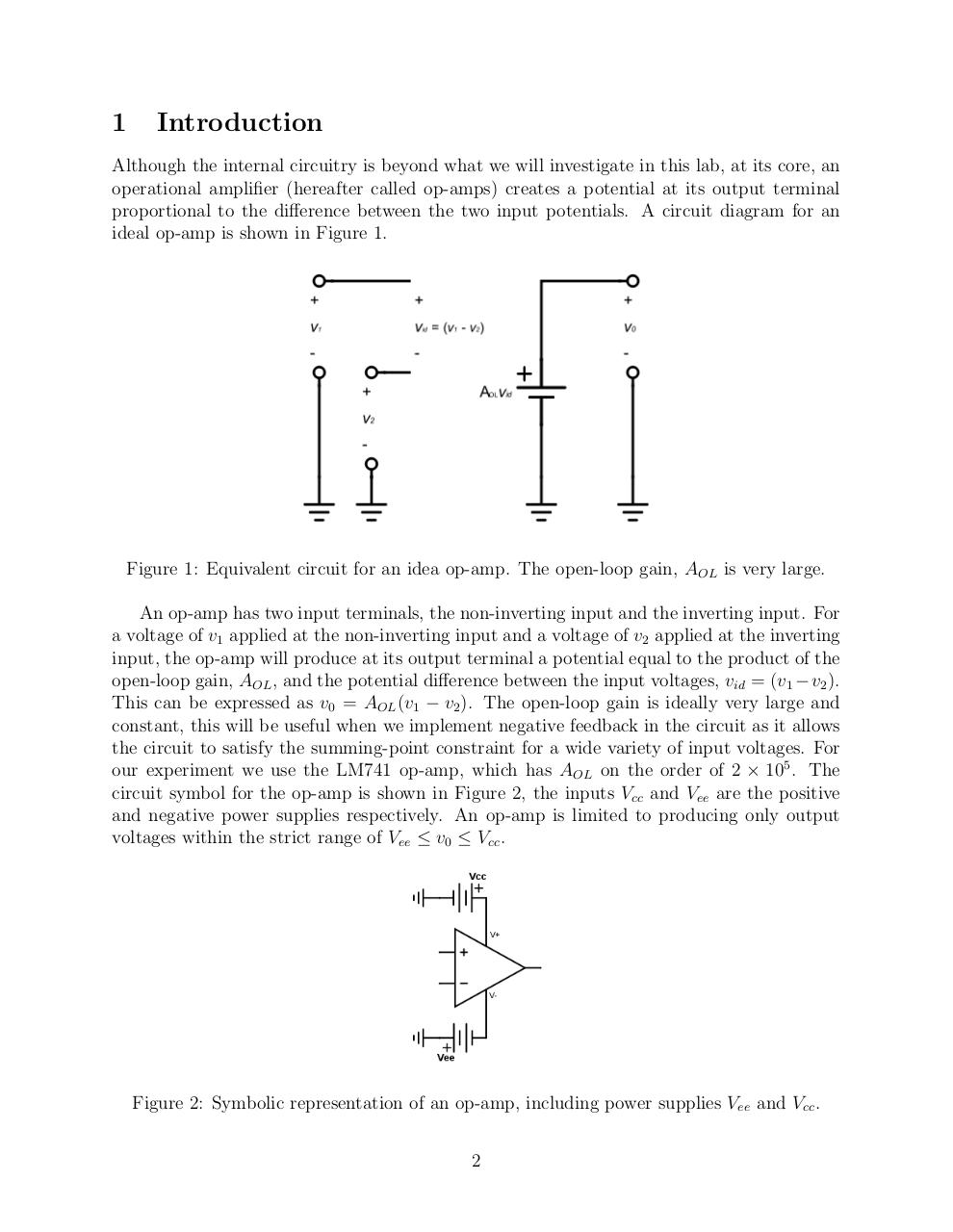# Op Amps.pdfPage 1 2 3 4 5 6 7 8 9 10 11 12 13

#### Text preview

1

Introduction

Although the internal circuitry is beyond what we will investigate in this lab, at its core, an
operational amplifier (hereafter called op-amps) creates a potential at its output terminal
proportional to the difference between the two input potentials. A circuit diagram for an
ideal op-amp is shown in Figure 1.

Figure 1: Equivalent circuit for an idea op-amp. The open-loop gain, AOL is very large.
An op-amp has two input terminals, the non-inverting input and the inverting input. For
a voltage of v1 applied at the non-inverting input and a voltage of v2 applied at the inverting
input, the op-amp will produce at its output terminal a potential equal to the product of the
open-loop gain, AOL , and the potential difference between the input voltages, vid = (v1 − v2 ).
This can be expressed as v0 = AOL (v1 − v2 ). The open-loop gain is ideally very large and
constant, this will be useful when we implement negative feedback in the circuit as it allows
the circuit to satisfy the summing-point constraint for a wide variety of input voltages. For
our experiment we use the LM741 op-amp, which has AOL on the order of 2 × 105 . The
circuit symbol for the op-amp is shown in Figure 2, the inputs Vcc and Vee are the positive
and negative power supplies respectively. An op-amp is limited to producing only output
voltages within the strict range of Vee ≤ v0 ≤ Vcc .

Figure 2: Symbolic representation of an op-amp, including power supplies Vee and Vcc .
2